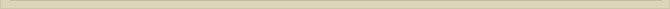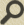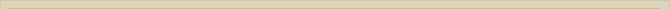## The photon

When you are interested in physics you must read “Unbelievable“!

To be able to explain the way the ether is functioning, much effort is spent on the assumed properties of the ether. Now we have a clear picture and a simple mathematical equation for the electron. The tremendous possibilities of the ether will soon become clear. It is commonly known that an electron can emit light. The electron is presented the equation for the total energy of the electron Wt: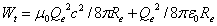When the dielectric displacement, while the charge –Qe was expanding, got the rotation speed c, the ceding of charge stopped. The joining point-volumes are no longer able to annihilate the charge –Qe at the sphere with radius Re. The expansion of charge Qe stopped.

When we assume the electron (Wt) has a speed in the surrounding ether the situation changes. In the chapter “The Lorentzfactor and the ether” we indicated that the accelerating force the electric field on charge Q declined with the factor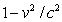, the Lorentz-factor in square. The reducing factor is here the Lorentz-factor in square because the force is conducted the profile of the ether still contributing and “in touch” with the moving particle.

In figure 18 the circle with radius Re presents the electron at rest. The total energy of the electron (Wt) is the intrinsic energy of the electron at rest (0 degrees Kelvin). When the electron moves in the ether with a speed v the spinning ether on the outside of the electron (Re) loses contact with the ether because the speed of the ether is now c+v. The outside of the electron loses contact with the ether. An expanding electron is not possible because the energy We will be less according to the equation when the electron expands. The radius Rv of the moving electron has to decline (Rv<Re) and will do so until the spinning ether of the electron has restored the contact with the surrounding ether.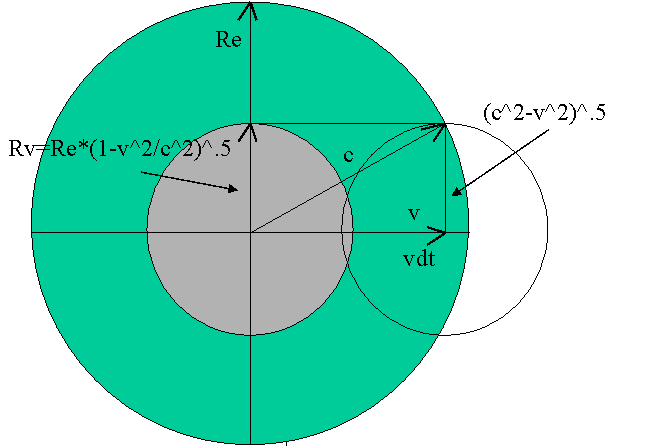Figure 18. The decline of the radius of the electron in motion.

The electron with speed v moves in dt seconds over a distance vdt. Because the ether lags with the reverse speed of light 1/c, the interaction between the electron and the surrounding ether diminishes with the Lorentz-factor to Rv. The electron can only decrease in size, because the charge is trapped the magnetic spin energy in the ether surrounding the electron.

The formula for Wt of the electron describes the enormous stability of the particle. The radius always adjusts adequately to the circumstances. The electron cannot disintegrate, because the energy is trapped. When the radius declines from Re to Rv, the energy of the electron increases with the Lorentz-factor. The total energy of the electron with speed v in the ether is Wv and according to the described process the energy is: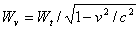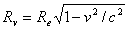According to the equivalence principle of mass and energy the mass Mv of the electron with speed v is: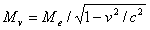When the electron is accelerated in an electric field in the ether the electron diminishes in size. When the electron leaves the electric field E the decreased electron is still in balance with the ether it travels in. The speed remains v and therefore the interaction between the ether and electron remains reduced with the Lorentz-factor and therefore the decreased size of the electron is maintained.

We consider now the situation wherein the electron is forced to a halt. The interacting with the ether now increases in reverse. The magnetic spin-energy did not alter during the acceleration. The electrostatic expansion force of the charge with radius Rv is, when the electron is halted, greater than the contraction force of the spin-energy (Re).

To make this situation clear we look at the total energy of the moving electron before it is forced to a halt: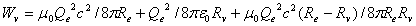The first part of the formula describes the magnetic spin energy of the electron at rest (Re). This energy is not affected when the electron is accelerated or slowed down. The second part is the electrostatic energy of the diminished electron (Rv). The third part is the magnetic energy induced in the ether due to the speed v.

To explain the oscillation of the electron when it is halted to a stop we have to consider an electron moving with speed v at rest with the ether. The potential energy is determined Rv and the magnetic spin energy Re.

When the electron accelerates the interaction with the ether decreases. The stability of the electron is guaranteed the magnetic energy existing in the ether surrounding the electron. The electron has to contract to Rv because the rotation energy is the same but the interacting of the charge with the ether decreased with Rv/Re. The magnetic rotation energy (Re) and the electrostatic energy (Rv) are in balance as long as the electron is moving in the ether with speed v. When the electron is forced to a halt the magnetic- and potential energy are no longer in equilibrium.

The interaction between electron and ether increases now with the Lorentz-factor. So when the electron is stopped the potential electrostatic energy is not in balance with the magnetic energy anymore. The electron will expand from Rv to Re to achieve equality between the expanding electrostatic force and the contracting spin force.

The electron expands before the lagging magnetic energy (the third part of the formula), caused the movement and the inert quality of ether reaches the electron. The equilibrium of electrostatic and magnetic spin energy is no more. The electron expands to Re; the energy level that coincides with the spin-energy. The electron is forced in a state of oscillation the surplus of electrostatic and magnetic energy. The vibrating electron interacts with the ether and induces an oscillating dielectric displacement in the ether around the electron. The electron is incredibly stable, so it is only a matter of time until the oscillating electron will reject the surplus of energy means of a photon.

The energy of the photon is given :

Ef=hc/λ

Where λ is the wavelength of the photon.

High-energy photons have enough magnetic and potential energy to separate the point-volume definitely in a positive and negative electron. When the high energy photon collides with a particle the kinetic resp. magnetic energy of the photon is transferred to the spin-energy of the positive and negative electron. The kinetic resp. magnetic energy of photon is transferred to the magnetic spin energy that traps the charges of the electrons.

The oscillation energy of the photon is transferred to the potential energy of both opposite charges. An electron couple is born (for further explanation we refer to the chapter The photon and the constant of Planck). Energy has become matter.

Next chapter: The photon mathematically